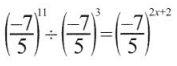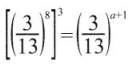# Grade 7 Exponents and Powers Worksheets

### Grade 7 Maths Exponents and Powers Multiple Choice Questions (MCQs)

1. Express 729 as a power of 3.
(a) 38
(b) 36
(c) 93
(d) none of these

2. The value of (-1)55 is:
(a) -1
(b) 1
(c) 0
(d) none of these

3. The value of (-1)500 is:
(a) -1
(b) 1
(c) 0
(d) none of these

4. Simplify and write in exponential form of 22 × 25
(a) 23
(b) 27
(c) 128
(d) none of these

5. Simplify and write in exponential form of (-4)100 × (-4)20.
(a) (-4)120
(b) (-4)80
(c) (-4)2000
(d) none of these

6. The exponent in the expression 37 is ……………. .
(a) 1
(b) 7
(c) 0
(d) 3

7. The value of 3° is ………….. .
(a) 0
(b) 3
(c) 1
(d) none of these

8. Multiplicative inverse of$\frac{1}{7}$ is …………… .
(a) 49
(b) 5
(c) 7
(d) -14

9. Fill in the Blank am ÷ an – a…….. Where m and n are natural numbers:-
(a) mn
(b) m + n
(c) m – n
(d) m ÷ n

10. Express (2a)4 in exponential form.
(a) 4a3
b) 16a4
(c) 2a4
(d) 8a4

11. In simplified form (3° + 4° + 5°)° is equals to:
(a) 12
(b) 3
(c) 12
(d) 1

12. The approximate distance of moon from the earth is 384, 467, 000 m and in exponential form this distance can be written as …………….. .
(a) 3.84, 467 × 108 m
(b) 384, 467 × 10-8 m
(c) 384, 467 × 10-9 m
(d) 3.844, 67 × 10-13 m

13. 7 × 10-5 m is the standard form of which of the following ……………. .
(a) 0.0007 m
(b) 0.000007 m
(c) 0.0000007 m
(d) 0.00007 m

14. Fill in the blank: (-1)even number = ……………… .
(a) 2 × (-1)
(b) 1
(c) 0
(d) -13

15. Fill in the blank: (-1)odd number = …………….. .
(a) 1
(b) -1
(c) 2
(d) 0

16. value of (3° + 2°) × 5° is
(a) 1
(b) 25
(c) 2
(d) 0

17. The Base in the expression 810 is …………….. .
(a) 10
(b) 2
(c) 8
(d) 800

18. Usual form of the expression 9 × 10-5 is given by …………….. .
(a) 0.00009
(b) 0.000009
(c) 90 × 10-4
(d) 0.09 × 10-3

19. 64 in exponential form is …………… .
(a) 26
(b) 162
(c)$\frac{1}{8^{2}}$
(d) 24

20. 1024 in exponential form is …………… .
(a) 26
(b) 162
(c)$\frac{1}{8^{2}}$
(d) none of these

### Grade 7 Maths Exponents and Powers Fill In The Blanks

1.2. (-3)3 × (-3)4 = …………… .
What power 2 is 32? ……………… .
4. Value of$\left[\left(\frac{2}{3}\right)^{2}\right]^{3}$ is …………….. .
5. The standard form of 2156000 is …………….. .

### Grade 7 Maths Exponents and Powers Match of column:

 Column I Column II 1. xm × xn a. xmn 2. xm ÷ xn b. 1 3. (xm)n c. (xy)n 4. xn × xn d. xm – n(m > n) 5. x ° e. xm + n

### Grade 7 Maths Exponents and Powers Very Short Answer Type Questions

Write in the standard form:

1. The distance between Earth and Moon is 384,000 km.
2. Speed of light in vacuum is 300,000,000 m/s.
3. 0.0034256
Find the value of:
4. 2° × 3° × 4°
5. (7° ÷ 30) × (8°- 5°)
6. 4° × 6° + 100°

a. Evaluate:

1. Find the value of x:2. Find the value of a:b. Find the value of x:

3. 5$\left\{\frac{2}{5}\right\}$ = 5x
4. (26 ÷ 2-3) × 214 = 2x

(b)$\frac{4.5 \times 10^{6}}{0.9 \times 10^{5}}$
(a)$\left(\frac{8}{9}\right)^{5} \times\left(\frac{9}{4}\right)$ = (2)m
(b) (7)3 ÷ (2)m =$\left(\frac{7}{2}\right)^{3}$